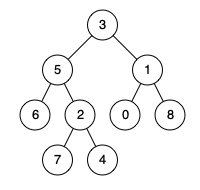Formatted question description: https://leetcode.ca/all/1676.html

# 1676. Lowest Common Ancestor of a Binary Tree IV

Medium

## Description

Given the root of a binary tree and an array of TreeNode objects nodes, return the lowest common ancestor (LCA) of all the nodes in nodes. All the nodes will exist in the tree, and all values of the tree’s nodes are unique.

Extending the definition of LCA on Wikipedia: “The lowest common ancestor of n nodes p_1, p_2, …, p_n in a binary tree T is the lowest node that has every p_i as a descendant (where we allow a node to be a descendant of itself) for every valid i”. A descendant of a node x is a node y that is on the path from node x to some leaf node.

Example 1:Input: root = [3,5,1,6,2,0,8,null,null,7,4], nodes = [4,7]

Output: 2

Explanation: The lowest common ancestor of nodes 4 and 7 is node 2.

Example 2:Input: root = [3,5,1,6,2,0,8,null,null,7,4], nodes = 

Output: 1

Explanation: The lowest common ancestor of a single node is the node itself.

Example 3:Input: root = [3,5,1,6,2,0,8,null,null,7,4], nodes = [7,6,2,4]

Output: 5

Explanation: The lowest common ancestor of the nodes 7, 6, 2, and 4 is node 5.

Example 4:Input: root = [3,5,1,6,2,0,8,null,null,7,4], nodes = [0,1,2,3,4,5,6,7,8] Output: 3 Explanation: The lowest common ancestor of all the nodes is the root node.

Constraints:**

• The number of nodes in the tree is in the range [1, 10^4].
• -10^9 <= Node.val <= 10^9
• All Node.val are unique.
• All nodes[i] will exist in the tree.
• All nodes[i] are distinct.

## Solution

Use depth first search. For each node, if it is in nodes, then the node is the lowest common ancestor in its subtree. Otherwise, check the node’s two children. If both subtrees contain nodes in nodes, then the current node is the lowest common ancestor in its subtree. If either subtree contains nodes in nodes, then the corresponding child is the lowest common ancestor in the current node’s subtree. Otherwise, return null, which indicates the current subtree does not contain any node in nodes. Finally, return the lowest common ancestor of all nodes in nodes.

/**
* Definition for a binary tree node.
* public class TreeNode {
*     int val;
*     TreeNode left;
*     TreeNode right;
*     TreeNode(int x) { val = x; }
* }
*/
class Solution {
public TreeNode lowestCommonAncestor(TreeNode root, TreeNode[] nodes) {
if (root == null || nodes == null || nodes.length == 0)
return null;
Set<TreeNode> set = new HashSet<TreeNode>();
for (TreeNode node : nodes)
return depthFirstSearch(root, set);
}

public TreeNode depthFirstSearch(TreeNode root, Set<TreeNode> set) {
if (root == null || set.contains(root))
return root;
TreeNode left = depthFirstSearch(root.left, set);
TreeNode right = depthFirstSearch(root.right, set);
if (left != null && right != null)
return root;
if (left != null)
return left;
if (right != null)
return right;
return null;
}
}